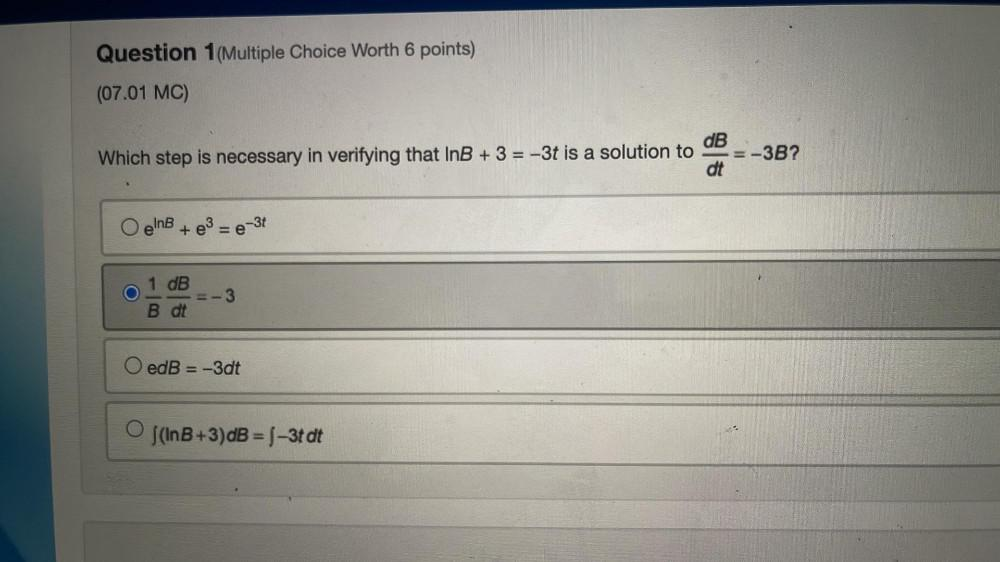Question:

# Which step is necessary in verifying that InB + 3 = -3t is a

Last updated: 7/26/2022Which step is necessary in verifying that InB + 3 = -3t is a solution to dB/dt=-3B? a)e^InB + e^3 = e^-3t b)1/B (dB/dt)=-3 c) edB = -3dt d)∫(InB+3)dB = ∫-3t dt